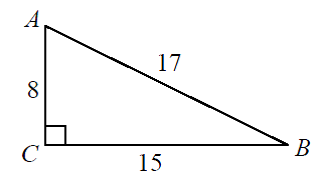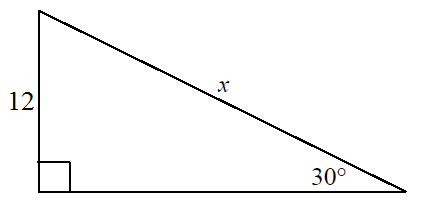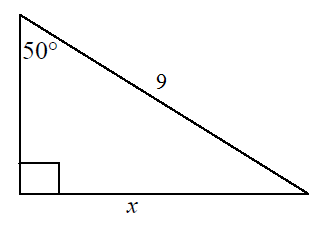# Use sine ratio to calculate angles and sides (Sin = $\frac{o}{h}$ )

### Use sine ratio to calculate angles and sides (Sin = $\frac{o}{h}$ )

One of the trigonometric ratios is sine ratio. It is the opposite side over the hypotenuse of right triangles. In other words, we can find the angles and sides of right-angled triangles by using the sine ratios.

#### Lessons

• Introduction
What are sine, cosine and tangent?

• 1.
Determine each sine ratio using a calculator
a)
$\sin 60^\circ$

b)
$\sin-60^\circ$

• 2.
Determine the angle to the nearest degree using a calculator
a)
$\sin \theta =0.57$

b)
$\sin \theta = -0.65$

• 3.
Determine the angles and sides using Sine ratio
a)
Find angle A and B:b)
Find the value of "x" using sinec)
Find the value of "x" using sine• 4.
Draw and label a right triangle to illustrate sine ratio, then calculate the angle.
a)
$\sin \theta = {5 \over 13}$